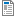National Curriculum

# Year 4/5 Maths Plans

Hamilton provide Mixed Year 4/5 weekly maths plans (below). We hope, in time, to develop maths blocks for this mixed year combination. Find out more about our plans to phase out these mixed age plans in favour of Year 4/5 maths blocks which we believe offer all the advantages of the weekly plans and more.

Supporting documents for set
• Week
• Title
1
+ Details
Place value

Year 4: Understand what each digit represents in a four-digit number. Write place value additions and subtractions. Order three-digit numbers, then four-digit numbers and place on a line. Year 5: Understand place value in five-digit numbers. Order 5-digit numbers. Add and subtract 1, 10, 100, 1000, 10,000. Place 4-digit numbers on a line and round to the nearest 10, 100 or 1000. Place 5-digit numbers on a line and round to the nearest 10, 100, 1000 or 10,000.Autumn Week 1 Plan
2
+ Details

Year 4: Mental addition of pairs of 2-digit numbers or 3-digit numbers and 2-digit numbers using place value or counting on. Mental subtraction of 2-digit numbers from 2-digit numbers and from numbers greater than 100 by counting back or counting up. Year 5: Written addition of money using column addition. Use using rounding to check answers. Understand place value in numbers with two decimal places. Mental division by 10 and 100 to give answers with two decimal places. Mental multiplication of numbers with 2 decimals by 10 and by 100. Place 2-place decimal numbers on a number line, compare and order them. Includes bar model examples.Autumn Week 2 Plan
3
+ Details

Year 4: Subtract by counting up, using Frog, check with addition. Add two, then three 3-digit numbers using compact written addition. Year 5: Find change from £20, £50 and £100 and find a difference between two amounts of money using counting up (Frog). Use place value to add and subtract. Add and subtract near multiples.  Use written addition to add pairs of 4-digit numbers then pairs of 5-digit numbers. Includes bar model examples.

4
+ Details
Shape

Year 4: Describe, name and sort 2D and 3D shapes. Describe, name and sort triangles. Use Venn diagrams or Carroll diagrams to sort shapes. Draw circles with different radii. Year 5: Identify, visualise and describe properties of 3D shapes, use these properties to sort 3D shapes. Recognise and describe properties of polygons. Classify quadrilaterals.

5
+ Details
Mental multiplication and division

Year 4: Double and halve 2-digit and 3-digit numbers using partitioning. Know 3, 4, 6, 8 times tables and associated division facts. Year 5: Use knowledge of times tables facts to help find common multiples. Find factors of two-digit numbers. Divide mentally, deciding whether to round up or down depending on the context. Use rules of divisibility. Find prime numbers less than 50.

6
+ Details
Number, fractions, written multiplication

Year 4: Use place value to add or subtract 4-digit numbers. Add and subtract 1, 10, 100, 1000 to and from 4-digit numbers. Year 5: Find equivalent fractions. Compare fractions with related denominators. Simplify fractions using factors. Revise using grid multiplication to multiply 3-digit numbers by single-digit numbers. Use short multiplication to multiply 3-digit numbers then 4-digit numbers by single-digit numbers. Includes bar model examples.

7
+ Details

Year 4: Add and subtract 3 or 4-digit numbers using place value. Add and subtract near multiples from a 3-digit number. Year 5: Understand place value in numbers with two decimal places. Count on and back in steps of 0.1 and 0.01. Add and subtract multiples of 0.1 or 0.01 without crossing multiples of 0.1 or 1. Mental subtraction to find a difference between a number with one or two decimal places and whole number by counting up (Frog). Subtract decimals with one or two decimal places by counting up from the smaller to the larger number (Frog). Includes bar model examples.

8
+ Details

Year 4: Subtract 3-digit numbers using expanded column subtraction (decomposition). Check subtraction with addition. Choose when to use counting up (Frog) and when to use column subtraction (decomposition). Year 5: Add pairs of five-digit numbers (five-digit answers). Use rounding to check. Use column subtraction (decomposition) to subtract pairs of four-digit numbers, three-digit numbers from four-digit numbers then pairs of five-digit numbers. Check subtraction with addition. Choose when to use counting up (Frog) and when to use column subtraction (decomposition).

9
+ Details
Time, measures and data

Year 4: Tell the time to the nearest minute. Use analogue, digital and Roman numeral clocks. Use am and pm times appropriately. Convert units of time, work out time intervals crossing the hour. Collect data and organise into bar charts and pictograms. Year 5: Convert between grams and kilograms, millilitres and litres. Convert between metres and kilometres. Know approximate conversions between common imperial units and metric units. Begin to draw line graphs and read intermediate points. Read timetables using the 24-hour clock.

10
+ Details
Multiplication and division

Year 4: Use grid method to multiply a 2-digit number by a 1-digit number. Use chunking on a number line to divide with no remainders. Year 5: Use short multiplication to multiply three-digit numbers by single-digit numbers including amounts of money, e.g. 3 × £4.56. Use a written method to divide numbers above the times tables. Round up or down after division according to the context. Use multiplication to check division.

11
+ Details
Division and fractions

Year 4: Divide 2-digit numbers by 1-digit numbers, just above the 10th multiple with remainders, using vertical written layout. Count ins 1/4s, 1/3s, 1/8s and 1/10s saying the equivalent fractions. Find unit fractions and non-unit fraction of amounts. Year 5: Write improper fractions as mixed numbers and vice versa. Compare and order fractions with related denominators. Add and subtract fractions with related denominators. Find fractions of amounts. Includes bar model examples.